# Solutions of Refraction of Light (Page No- 252) - Physics By Lakhmir Singh, Class 10 Class 10 Notes | EduRev

## Class 10 : Solutions of Refraction of Light (Page No- 252) - Physics By Lakhmir Singh, Class 10 Class 10 Notes | EduRev

The document Solutions of Refraction of Light (Page No- 252) - Physics By Lakhmir Singh, Class 10 Class 10 Notes | EduRev is a part of the Class 10 Course Class 10 Physics Solutions By Lakhmir Singh & Manjit Kaur.
All you need of Class 10 at this link: Class 10

Lakhmir Singh Physics Class 10 Solutions Page No:252

Question 3: Which type of lenses are :
(a) thinner in the middle than at the edges ?
(b) thicker in the middle than at the edges ?

Solution : (a) Concave lenses.
(b) Convex lenses.

Question 4: A ray of light is going towards the focus of a concave lens. Draw a ray diagram to show the path of this ray of light after refraction through the lens.

Solution :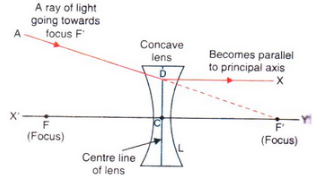Ray of light going towards the focus of a concave lens.

Question 5: (a) What type of images can a convex lens make ?
(b) What type of image is always made by a concave lens ?

Solution : (a) Real and virtual.
(b) Virtual.

Question 6: Take down this figure into your answer book and complete the path of the ray.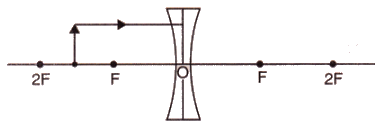Solution :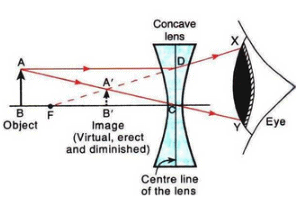Question 7: Fill in the following blanks with suitable words :
(a) A convex lens……. rays of light, whereas a concave lens……….. rays of light.
(b) Lenses refract light to form images : a……… lens can form both real and virtual images, but a diverging
lens forms only……….. images.

Solution : (a) converges; diverges
(b) converging; virtual

Question 8: Things always look small on viewing through a lens. What is the nature of the lens ?

Solution : Concave lens.

Question 9: An object lies at a distance of 2ƒ from a concave lens of focal length ƒ. Draw a ray-diagram to illustrate the image formation.

Solution :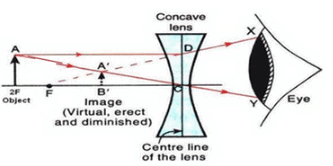Question 10: Show by drawing a ray-diagram that the image of an object formed by a concave lens is virtual, erect and diminished.

Solution :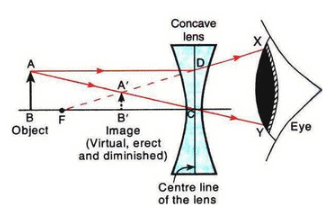Question 11: Give the position, size and nature of image formed by a concave lens when the object is placed :
(a) anywhere between optical centre and infinity.
(b) at infinity.

Solution : (a) When the object is placed anywhere between optical centre and infinity, the image is formed between optical centre and focus. It is diminished, virtual and erect.
(b) When the object is placed at infinity, the image is formed at focus. It is highly diminished, virtual and erect.

Question 12: Which type of lens is : (a) a converging lens, and which is (b) a diverging lens ? Explain your answer with diagrams.

Solution : (a) A convex lens is a converging lens because it converges a parallel beam of light rays passing through it at its focus.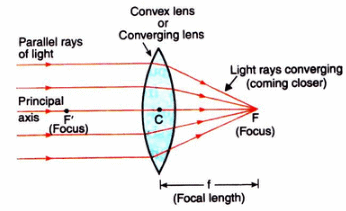Figure — A convex lens converges (brings closer) a parallel beam of light rays to a point F on its other side (right side).

(b) A concave lens is a diverging lens because it diverges the parallel beam of rays passing through it.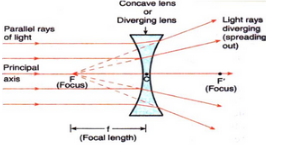Figure — A concave lens diverge  (spreads out) a parallel beam of light rays.

Question 13: With the help of a diagram, explain why the image of an object viewed through a concave lens appears smaller and closer than the object.

Solution : (a) Smaller.
(b) Bigger.
Image is virtual in both the cases.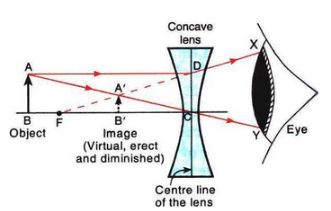As shown by the diagram, the image of an object viewed through a concave lens appears smaller and closer than the object.

Question 14: How would a pencil look like if you saw it through (a) a concave lens, and (b) a convex lens ? (Assume the pencil is close to the lens). Is the image real or virtual ?

Solution :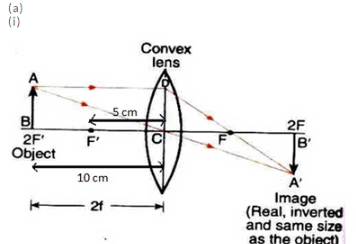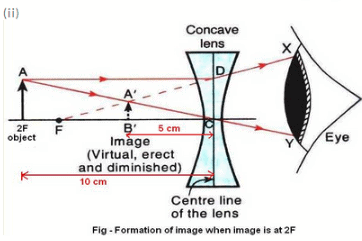(b) Use of convex mirror: As rear-view mirror in vehicles
Use of concave mirror: As shaving mirrors
Use of convex lens: For making simple camera
Use of concave lens: As eye-lens in Galilean telescope

Question 15: (a) An object is placed 10 cm from a lens of focal length 5 cm. Draw the ray diagrams to show the formation
of image if the lens is (i) converging, and (ii) diverging.
(b) State one practical use each of convex mirror, concave mirror, convex lens and concave lens.

Solution :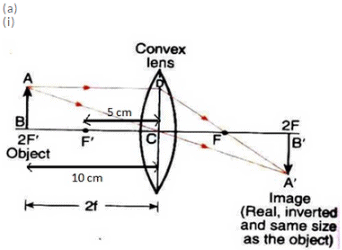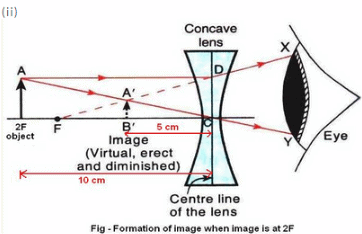(b) Use of convex mirror: As rear view mirror in vehicles
Use of concave mirror: As shaving mirrors
Use of convex lens: For making simple camera
Use of concave lens: As eye-lens in Galilean telescope

Question 16: (a) Construct ray diagrams to illustrate the formation of a virtual image using (i) a converging lens, and
(ii) a diverging lens.
(b) What is the difference between the two images formed above ?

Solution :(a)

(i) Formation of virtual image using a converging lens: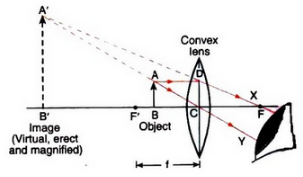(ii) Formation of virtual image using a diverging lens;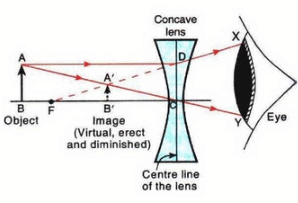(b) The virtual image formed by a converging lens is magnified whereas that formed by a diverging lens is diminished.

Offer running on EduRev: Apply code STAYHOME200 to get INR 200 off on our premium plan EduRev Infinity!

94 docs

,

,

,

,

,

,

,

,

,

,

,

,

,

,

,

,

,

,

,

,

,

,

,

,

;